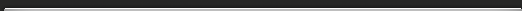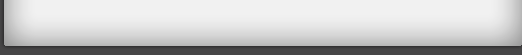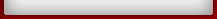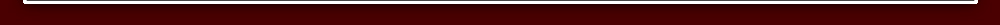Tutorial 2

## Tutorial 2

Using VERTICALLY AND CROSSWISE you do not need the multiplication tables beyond 5 X 5.

Suppose you need 8 x 7 8 is 2 below 10 and 7 is 3 below 10. Think of it like this:The diagram below shows how you get it.You subtract crosswise 8-3 or 7 - 2 to get 5, the first figure of the answer. And you multiply vertically: 2 x 3 to get 6, the last figure of the answer.
That's all you do:

See how far the numbers are below 10, subtract one number's deficiency from the other number, and multiply the deficiencies together.
7 x 6 = 42Here there is a carry: the 1 in the 12 goes over to make 3 into 4.

Exercise 1 Tutorial 2
Multply These:
1) 8 x 8 =
2) 9 x 7 =
3) 8 x 9 =
4) 7 x 7 =
5) 9 x 9 =
6) 6 x 6 =

### Multiplying numbers just Below 100.

Suppose you want to multiply 88 by 98. Not easy,you might think. But with VERTICALLY AND CROSSWISE you can give the answer immediately, using the same method as above
Both 88 and 98 are close to 100. 88 is 12 below 100 and 98 is 2 below 100.
You can imagine the sum set out like this:As before the 86 comes from subtracting crosswise: 88 - 2 = 86 (or 98 - 12 = 86: you can subtract either way, you will always get the same answer). And the 24 in the answer is just 12 x 2: you multiply vertically.
So 88 x 98 = 8624

Exercise 2 Tutorial 2

This is so easy it is just mental arithmetic.

Try some:

1) 87 x 98 =
2) 88 x 97 =
3) 77 x 98 =
4) 93 x 96 =
5) 94 x 92 =
6) 64 x 99 =
7) 98 x 97 =

### Multiplying numbers just over 100.

103 x 104 = 10712
The answer is in two parts: 107 and 12,
107 is just 103 + 4 (or 104 + 3),
and 12 is just 3 x 4.

Similarly 107 x 106 = 11342
107 + 6 = 113 and 7 x 6 = 42

Exercise 3 Tutorial 2

Again, just for mental arithmetic
Try a few:

1) 102 x 107 =
2) 106 x 103 =
3) 104 x 104 =
4) 109 x 108 =
5) 101 x123 =
6) 103 x102 =